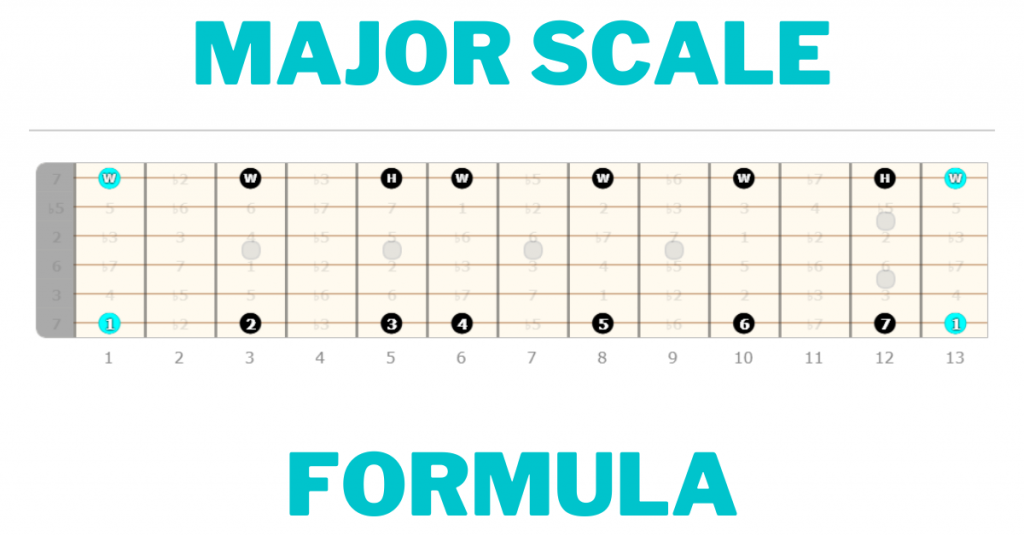# Major Scale Formula Definition (Guitar)## Understanding the Major Scale Formula

Understanding the major scale formula boils down to knowing the degrees and steps that make up a major scale.

### What are the 7 notes of a major scale?

The 7 notes/scale degrees of a major scale are: 1-2-3-4-5-6-7

Each of these degrees represents a distance from the root note. These distances are called intervals.

For example, if we take the major scale formula and apply it to the C Note we’ll get:

C-D-E-F-G-A-B

### What are the steps in a major scale?

The major scale, like any scale, can be visualized using a series of whole and half steps.

There are 7 total steps in the major scale. They are, starting from the root note:

Whole-Whole-Half-Whole-Whole-Whole-Half

This can also be abbreviated as: W-W-H-W-W-W-H

Using this series of Whole and Half steps, pick a root note on a single string and follow the steps.

For example, if you start on the 1st fret of the Low E string, which is an F note, you’ll end up with an F major scale.